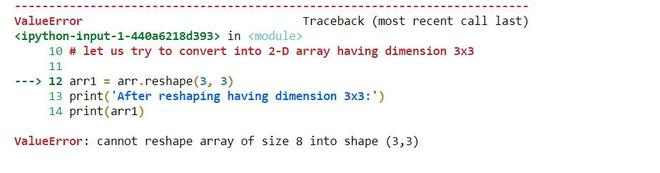# Convert a 1D array to a 2D Numpy array

• Difficulty Level : Easy
• Last Updated : 13 Jan, 2021

Numpy is a Python package that consists of multidimensional array objects and a collection of operations or routines to perform various operations on the array and processing of the array. This package consists of a function called numpy.reshape which is used to convert a 1-D array into a 2-D array of required dimensions (n x m).  This function gives a new required shape without changing the data of the 1-D array.

Syntax: numpy.reshape(array, new_shape, order)

Parameters:

•  array: is the given 1-D array that will be given a new shape or converted into 2-D array
• new_shape: is the required shape or 2-D array having int or tuple of int
• order: ‘C’ for C style, ‘F’ for Fortran style, ‘A’ if data is in Fortran style then Fortran like order else C style.

Examples 1:

## Python3

 `import` `numpy as np``# 1-D array having elements [1 2 3 4 5 6 7 8]``arr ``=` `np.array([``1``, ``2``, ``3``, ``4``, ``5``, ``6``, ``7``, ``8``])`` ` `# Print the 1-D array``print` `(``'Before reshaping:'``)``print` `(arr)``print` `(``'\n'``)`` ` `# Now we can convert this 1-D array into 2-D in two ways`` ` `# 1. having dimension 4 x 2``arr1 ``=` `arr.reshape(``4``, ``2``)``print` `(``'After reshaping having dimension 4x2:'``)``print` `(arr1)``print` `(``'\n'``)`` ` `# 2. having dimension 2 x 4``arr2 ``=` `arr.reshape(``2``, ``4``)``print` `(``'After reshaping having dimension 2x4:'``)``print` `(arr2)``print` `(``'\n'``)`

Output:

```Before reshaping:
[1 2 3 4 5 6 7 8]
After reshaping having dimension 4x2:
[[1 2]
[3 4]
[5 6]
[7 8]]
After reshaping having dimension 2x4:
[[1 2 3 4]
[5 6 7 8]]```

Example 2: Let us see an important observation that whether we can reshape a 1-D array into any 2-D array.

## Python3

 `import` `numpy as np``# 1-D array having elements [1 2 3 4 5 6 7 8]``arr ``=` `np.array([``1``, ``2``, ``3``, ``4``, ``5``, ``6``, ``7``, ``8``])`` ` `# Print the 1-D array``print``(``'Before reshaping:'``)``print``(arr)``print``(``'\n'``)`` ` `# let us try to convert into 2-D array having dimension 3x3``arr1 ``=` `arr.reshape(``3``, ``3``)``print``(``'After reshaping having dimension 3x3:'``)``print``(arr1)``print``(``'\n'``)`

Output:This concludes that the number of elements should be equal to the product of dimension i.e. 3×3=9 but total elements = 8;

Example 3: Another example is that we can use the reshape method without specifying the exact number for one of the dimensions. Just pass -1 as the value and NumPy will calculate the number.

## Python3

 `import` `numpy as np``# 1-D array having elements [1 2 3 4 5 6 7 8]``arr ``=` `np.array([``1``, ``2``, ``3``, ``4``, ``5``, ``6``, ``7``, ``8``])`` ` `# Print the 1-D array``print``(``'Before reshaping:'``)``print``(arr)``print``(``'\n'``)`` ` ` ` `arr1 ``=` `arr.reshape(``2``, ``2``, ``-``1``)``print``(``'After reshaping:'``)``print``(arr1)``print``(``'\n'``)`

Output:

```Before reshaping:
[1 2 3 4 5 6 7 8]
After reshaping:
[[[1 2]
[3 4]]
[[5 6]
[7 8]]]```

My Personal Notes arrow_drop_up